# AGC & EEP: Denmark

#### unmerged(40707)

##### Just call me Yoda in private!
Shouldn't reworked SWE_291021 check context? Is there a point for this event if Sweden owns Gotland or Denmark doesn't exist (and maybe check of any event in the sequence just before)?

Reworked twin SWE_291022 / SWE_291023 (including proposed changes):
Code:
``````#(1449-1459) The invasion of Gotland is launched -I-
event = {
id = 291022
trigger = {
atwar = no
ai = yes
event = 291021 #SWE: Erik has lost support from Denmark
NOT = {
owned = { province = 308 data = -1 } #Gotland
owned = { province = 308 data = POM } #Gotland
}
}
random = no
country = SWE
name = "EVENTNAME291022" #The invasion of Gotland is launched
desc = "EVENTHIST291022"
#-#In 1449 the Swedish forces tried to invade Gotland.

date = { day = 1 month = january year = 1449 }
offset = 90
deathdate = { day = 1 month = january year = 1459 }

action_a = {
name = "ACTIONNAME291022A" #Launch the invasion of Gotland
command = { type = stability value = -1 }
command = { type = desertion which = -1 value = 5000 }
command = { type = sleepevent which = 291023 } #SWE: The invasion of Gotland is launched -II-
}
}
#(1449-1459) The invasion of Gotland is launched -II-
event = {
id = 291023
trigger = {
atwar = no
ai = yes
event = 291021 #SWE: Erik has lost support from Denmark
owned = { province = 308 data = POM } #Gotland
}
random = no
country = SWE
name = "EVENTNAME291022" #The invasion of Gotland is launched
desc = "EVENTHIST291022"
#-#In 1449 the Swedish forces tried to invade Gotland.

date = { day = 1 month = january year = 1449 }
offset = 90
deathdate = { day = 1 month = january year = 1459 }

action_a = {
name = "ACTIONNAME291022A" #Launch the invasion of Gotland
command = { type = stability value = -1 }
command = { type = desertion which = -1 value = 5000 }
command = { type = trigger which = 259008 } #POM: The invasion of Gotland
command = { type = sleepevent which = 291022 } #SWE: The invasion of Gotland is launched -I-
}
}``````

I'm not sure I understand the proposed changes for Random_338011 and DAN_159049.
338011 was intended to be random while GOT was a existing country. Mentioned provinces in the trigger are the ones that will be targetted but, of course not if owned by POM now. This event shouldn't fire for any other country not owning at least one of the provinces. Why removing all the province conditions in the trigger? IMHO, removal of ownership by GOT is sufficient.
About dates, a random event can't have dates. Replace them with two conditions:
Code:
``````		year = 1439
NOT = { year = 1448 }``````
DAN_159049 was intended only for the end of this random event. I don't understand why it trigger 338011 now. Maybe trigger of DAN_159049 should check if Gotland is not owned or simply not controlled by Pomerania instead. In this case, plundering can no longer happen. Maybe DAN_159049 should be a provincespec event for Gotland province and not a Danish one.

I'm not sure now, is there a chance for Pomerania to get Gotland province by event (ceded by Denmark?) as part of the sequence now?

#### Toio

##### Field Marshal
•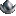•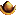•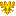•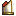••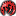YodaMaster said:
Shouldn't reworked SWE_291021 check context? Is there a point for this event if Sweden owns Gotland or Denmark doesn't exist (and maybe check of any event in the sequence just before)?

Reworked twin SWE_291022 / SWE_291023 (including proposed changes):
Code:
``````#(1449-1459) The invasion of Gotland is launched -I-
event = {
id = 291022
trigger = {
atwar = no
ai = yes
event = 291021 #SWE: Erik has lost support from Denmark
NOT = {
owned = { province = 308 data = -1 } #Gotland
owned = { province = 308 data = POM } #Gotland
}
}
random = no
country = SWE
name = "EVENTNAME291022" #The invasion of Gotland is launched
desc = "EVENTHIST291022"
#-#In 1449 the Swedish forces tried to invade Gotland.

date = { day = 1 month = january year = 1449 }
offset = 90
deathdate = { day = 1 month = january year = 1459 }

action_a = {
name = "ACTIONNAME291022A" #Launch the invasion of Gotland
command = { type = stability value = -1 }
command = { type = desertion which = -1 value = 5000 }
command = { type = sleepevent which = 291023 } #SWE: The invasion of Gotland is launched -II-
}
}
#(1449-1459) The invasion of Gotland is launched -II-
event = {
id = 291023
trigger = {
atwar = no
ai = yes
event = 291021 #SWE: Erik has lost support from Denmark
owned = { province = 308 data = POM } #Gotland
}
random = no
country = SWE
name = "EVENTNAME291022" #The invasion of Gotland is launched
desc = "EVENTHIST291022"
#-#In 1449 the Swedish forces tried to invade Gotland.

date = { day = 1 month = january year = 1449 }
offset = 90
deathdate = { day = 1 month = january year = 1459 }

action_a = {
name = "ACTIONNAME291022A" #Launch the invasion of Gotland
command = { type = stability value = -1 }
command = { type = desertion which = -1 value = 5000 }
command = { type = trigger which = 259008 } #POM: The invasion of Gotland
command = { type = sleepevent which = 291022 } #SWE: The invasion of Gotland is launched -I-
}
}``````

i still do not like this event, whats it all for?

I'm not sure I understand the proposed changes for Random_338011 and DAN_159049.
338011 was intended to be random while GOT was a existing country. Mentioned provinces in the trigger are the ones that will be targetted but, of course not if owned by POM now. This event shouldn't fire for any other country not owning at least one of the provinces. Why removing all the province conditions in the trigger? IMHO, removal of ownership by GOT is sufficient.

Removing of ownership of GOT was my initial thoughts, but the issue is Erik residing in Gotland from 1440 supplemented his income by supporting piracy.

About dates, a random event can't have dates. Replace them with two conditions:
Code:
``````		year = 1439
NOT = { year = 1448 }``````
DAN_159049 was intended only for the end of this random event. I don't understand why it trigger 338011 now. Maybe trigger of DAN_159049 should check if Gotland is not owned or simply not controlled by Pomerania instead. In this case, plundering can no longer happen. Maybe DAN_159049 should be a provincespec event for Gotland province and not a Danish one.

unsure on this

I'm not sure now, is there a chance for Pomerania to get Gotland province by event (ceded by Denmark?) as part of the sequence now?

yes , POM get gotland with DAN event 159048 in 1440

#### unmerged(40707)

##### Just call me Yoda in private!
What about SWE_291021 then? Any idea for a trigger?

Toio said:
i still do not like this event, whats it all for?
Why not removing them then... if they are non sense?

Toio said:
Removing of ownership of GOT was my initial thoughts, but the issue is Erik residing in Gotland from 1440 supplemented his income by supporting piracy

unsure on this
In this case, here is my proposal (based on 1.57 beta 14):
Code:
``````#(1440-1447) Erik inherits Gotland for Pomerania
event = {
id = 159048
trigger = {
owned = { province = 308 data = -1 } #Gotland
[COLOR=Yellow]exists = POM[/COLOR]
}
random = no
country = DAN
name = "EVENTNAME159048" #Erik takes Gotland
desc = "EVENTHIST159048"
#-#

date = { day = 1 month = march year = 1440 }
offset = 120
deathdate = { day = 30 month = december year = 1447 }

action_a = {
name = "ACTIONNAME159048A" #Pomerania rules Gotland
command = { type = secedeprovince which = POM value = 308 } #Gotland
}
}``````
#-#In 1440, Erik, having been deposed in Denmark and Sweden, was succeeded by his nephew, Christopher of Bavaria, who had been chosen for the thrones. After he had been deposed as king in Sweden and Denmark, the Norwegian Riksråd remained loyal to him, and wanted him to remain king of Norway only. Erik left Denmark and settled at his castle Visborg in Gotland. In 1448, Erik handed over Gotland to Denmark in return for the permission to leave for Pomerania.

Code:
``````#(1419-1459) Merchants are being plundered - Erik VII sequence - Random
event = {
id = 338011
trigger = {
owned = { province = 308 data = POM } #Gotland
control = { province = 308 data = POM } #Gotland
year = 1439
NOT = { year = 1448 }
OR = {
AND = {
owned = { province = 307 data = -1 } #Sjælland
NOT = { owned = { province = 307 data = POM } }
}
AND = {
owned = { province = 251 data = -1 } #Skåne
NOT = { owned = { province = 251 data = POM } }
}
AND = {
owned = { province = 252 data = -1 } #Småland
NOT = { owned = { province = 252 data = POM } }
}
AND = {
owned = { province = 254 data = -1 } #Svealand
NOT = { owned = { province = 254 data = POM } }
}
AND = {
owned = { province = 1469 data = -1 } #Gästrikland
NOT = { owned = { province = 1469 data = POM } }
}
AND = {
owned = { province = 342 data = -1 } #Finland
NOT = { owned = { province = 342 data = POM } }
}
AND = {
owned = { province = 265 data = -1 } #Nyland
NOT = { owned = { province = 265 data = POM } }
}
AND = {
owned = { province = 275 data = -1 } #Ingermanland
NOT = { owned = { province = 275 data = POM } }
}
AND = {
owned = { province = 276 data = -1 } #Estland
NOT = { owned = { province = 276 data = POM } }
}
AND = {
owned = { province = 282 data = -1 } #Livland
NOT = { owned = { province = 282 data = POM } }
}
AND = {
owned = { province = 283 data = -1 } #Kurland
NOT = { owned = { province = 283 data = POM } }
}
AND = {
owned = { province = 289 data = -1 } #Memel
NOT = { owned = { province = 289 data = POM } }
}
AND = {
owned = { province = 290 data = -1 } #Prussia
NOT = { owned = { province = 290 data = POM } }
}
AND = {
owned = { province = 301 data = -1 } #Danzig
NOT = { owned = { province = 301 data = POM } }
}
AND = {
owned = { province = 302 data = -1 } #Hinterpommern
NOT = { owned = { province = 302 data = POM } }
}
AND = {
owned = { province = 304 data = -1 } #Vorpommern
NOT = { owned = { province = 304 data = POM } }
}
AND = {
owned = { province = 305 data = -1 } #Mecklenburg
NOT = { owned = { province = 305 data = POM } }
}
}
}
random = yes
name = "EVENTNAME338011" #Merchants are being plundered
desc = "EVENTHIST338011"
#-#

action_a = {
name = "ACTIONNAME338011A" #This must come to an end!
command = { type = stability value = -1 }
command = { type = merchants value = -1 }
command = { type = ADM which = -2 value = 6 }
command = { type = trade value = -50 }
command = { type = relation which = POM value = -50 }
command = { type = casusbelli which = POM value = 12 }
}
}``````
#-#Our merchants are being plundered by a pirate fleet operating out of Gotland under the command of Erik of Pomerania.

Code:
``````#(1441-1448) The invasion of Gotland
event = {
id = 159049
trigger = {
owned = { province = 308 data = POM } #Gotland
}
random = no
country = DAN
name = "EVENTNAME259008" #The invasion of Gotland
desc = "EVENTHIST159049"
#-#

date = { day = 11 month = may year = 1441 }
offset = 10
deathdate = { day = 29 month = december year = 1448 }

action_a = {
name = "ACTIONNAME159049A" #Gotland is yet again under Danish rule
command = { type = stability value = 1 }
command = { type = sleepevent which = 338011 } #Random National: Merchants are being plundered
}
}``````
#-#In 1439 the Danish forces overrun and annex Gotland. Gotland agrees to Danish rule and everlasting peace under Erik.

Not sure if EVENTHIST159049 makes sense now. Could it be reworked?

Toio said:
yes , POM get gotland with DAN event 159048 in 1440
Ok, sequence with removed SWE_291022 and SWE_291023 + reworked EVENTHIST159049 should be fine now.

But there is still a consistency issue (dates) with this event:
Code:
``````#(1449-1459) The invasion of Gotland
event = {
id = 259008
trigger = {
owned = { province = 308 data = -1 }
event = 291021 #SWE: Erik has lost support from Denmark
}
random = no
country = POM
name = "EVENTNAME259008" #The invasion of Gotland
desc = "EVENTHIST259008"
#-#

date = { day = 1 month = january year = 1449 }
offset = 10
deathdate = { day = 1 month = january year = 1459 }

action_a = {
name = "ACTIONNAME259008A" #Return control of Gotland to Denmark
command = { type = secedeprovince which = DAN value = 308 } #Gotland
command = { type = removecore which = 308 } #Gotland
command = { type = trigger which = 291024 } #SWE: The invasion of Gotland
[COLOR=DeepSkyBlue]command = { type = trigger which = 159049 } #DAN: The invasion of Gotland[/COLOR]
command = { type = sleepevent which = 291025 } #SWE: Gotland is yet again in Danish hands
}
}``````
#-#In 1449 the Swedish forces in Gotland aided Erik in the war against the Danes. While Erik was successful in defending the island, he realised that there was no way he could win the war. Therefore he agreed to leave Gotland to the Danes for safe passage to Pomerania.

Last edited:

#### Toio

##### Field Marshal
••••••@ yoda ..........all ok with your events

Code:
``````#(1419-1459) Erik has lost support from Denmark
event = {
id = 291021 [COLOR=Red]#triggered by GOT_367001[/COLOR]
random = no
country = SWE
name = "EVENTNAME291021" #[COLOR=Red]Erik has lost support from Denmark[/COLOR] [COLOR=Yellow]Sweden claims Gotland and Norway[/COLOR]
desc = "EVENTHIST291021"

[COLOR=Yellow]date = { day = 27 month = june year = 1448 }
offset = 90
deathdate = { day = 1 month = january year = 1459 }[/COLOR]

action_a = {
name = "ACTIONNAME291021A" #Gotland belongs to Sweden
command = { type = addcore which = 308 } #Gotland
command = { type = relation which = [COLOR=Red]GOT[/COLOR] [COLOR=Yellow]DAN [/COLOR]value = -400 }
}
}``````
#-#When Erik retreated to Gotland and began pirating on merchants in the Baltic Sea he lost support from Denmark. An opportunity to seize the claim on Gotland for the Swedish nobles presented itself at the death of Christopher of Bavaria in 1448, without a direct heir, Charles VIII was elected king of Sweden on June 20 and on June 28 he was hailed as the new monarch at the Stone of Mora, not far from Uppsala. Charles now claimed Norway and Gotland as Swedish crown lands. His election as king of Sweden resulted in an effort to reestablish the Kalmar Union, this time under Swedish initiative, and in 1449 he was also elected king of Norway. The Danish had in September 1448 elected Christian I as their new monarch. Soon, in 1450, Charles was forced to cede Norway to Christian. From 1451, Sweden and Denmark were in state of war against each other which would last for 3 years.
.........................................................................

#### unmerged(40707)

##### Just call me Yoda in private!
Ok, do we remove SWE_291022 and SWE_291023 then?

And what about EVENTHIST159049 and trigger command(s) in POM_259008?

#### Toio

##### Field Marshal
••••••YodaMaster said:
Ok, do we remove SWE_291022 and SWE_291023 then?

still trying to figure out what the original modder intended. I will keep you posted

And what about EVENTHIST159049 and trigger command(s) in POM_259008?

159049..............I think we get rid of it

259008..............is needed with some modifications

#### unmerged(40707)

##### Just call me Yoda in private!
Toio said:
159049..............I think we get rid of it
What do you mean, according to post #103?

#### Toio

##### Field Marshal
••••••YodaMaster said:
What do you mean, according to post #103?

the best I think for this event is

#(1448-1458) The invasion of Gotland
event = {
id = 159049
trigger = {
owned = { province = 308 data = POM } #Gotland
}
random = no
country = DAN
name = "EVENTNAME259008" #The invasion of Gotland
desc = "EVENTHIST159049"
#-#

date = { day = 11 month = may year = 1441 1448 }
offset = 10
deathdate = { day = 29 month = december year = 1448 1458 }

action_a = {
name = "ACTIONNAME159049A" #Gotland is yet again under needs Danish rule
command = { type = stability value = 1 }
command = { type = addcore which = 308 } #Gotland
command = { type = sleepevent which = 338011 } #Random National: Merchants are being plundered
}
}

text , needs to be redone

#### unmerged(40707)

##### Just call me Yoda in private!
Fine, SWE_291022 and SWE_291023 are not a "problem" per se now. Only remaining problem I'm aware of is POM_259008 and the triggering of events (if not solved with post above)... and EVENTHIST159049.

Removal of GOT tag was not so easy as it seemed to be.#### unmerged(84806)

##### Lt. General
•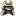•I was playing Norway (AGCEEP v1.55), and had annexed Denmark, so I was in possession of Sjælland. Event 244002 fired, where you can select to: A become Denmark and rule from Copenhagen, B become Denmark and rule from Oslo, C release Denmark as vassal (I'm not quite sure why this option is included - why do you want to release Denmark when you just recently have annexed them?) and finally D status quo (adds some cores on Danish lands).

Option B and D is nearly identical, except for two things. In B you get the Danish flag, and their monarchs and leaders. In D you keep the Norwegian flag. In other words option D is quite useless compared to option B. However, I really would like to keep on playing as Norway, or rather with the Norwegian flagD). Is it possible to edit the event so Norway will inherit the Danish leaders? I know that this is very ahistorical, but then again, the whole event is rather ahistorical. I hope I made my point clear... so, what do you think about it?

#### unmerged(37697)

##### Banned
Olav said:
...Is it possible to edit the event so Norway will inherit the Danish leaders? I know that this is very ahistorical, but then again, the whole event is rather ahistorical. I hope I made my point clear... so, what do you think about it?
Yeah, I suggest you do so (if you know how) and post the sequence here for us to see. Remember to use Norwegian leader IDs, not Danish ones.

It's the same as the alt. Iberia or the kingdom of Germany sequence - another country tag getting someone else's leaders.

If you feel it makes sense and would make any form of positive addition to the AGCEEP (which I feel this sequence would), then feel free to have a shot.#### unmerged(84806)

##### Lt. General
••Yeah, I gave it a shot. I copied over the Danish leaders to the NOR leader-file and gave them new IDs and set dormant = yes. I also added the wakeleader command to the event-file. However when I annexed Denmark, I never got any of the Danish leaders (I just discovered that I had an extra bracket in the leaderfile: Doh!). So I fixed that now, started a new game as Norway and saved. All the Danish leaders are there now, so hopefully it will work (but I haven't tested it yet!).

Code:
``````#(1419-1820) Ascendance to the Danish throne
event = {
id = 244002
trigger = {
owned = { province = 307 data = -1 }
control = { province = 307 data = -1 }
NOT = { exists = DAN }
}
random = no
country = NOR
name = "EVENTNAME244002" #Ascendance to the Danish throne
desc = "EVENTHIST244002"
#-#

date = { day = 1 month = january year = 1419 }
offset = 360
deathdate = { year = 1820 }

action_a = {
name = "ACTIONNAME244002A" #Claim the Danish throne and rule from Copenhagen
command = { type = stability value = -2 }
command = { type = flagname which = "" }
command = { type = country which = DAN }
command = { type = capital which = 307 } #Sjælland
command = { type = domestic which = aristocracy value = 2 }
command = { type = domestic which = centralization value = -1 }
command = { type = domestic which = innovative value = -1 }
command = { type = addcore which = 307 } #Sjælland
command = { type = addcore which = 308 } #Gotland
command = { type = addcore which = 309 } #Jylland
command = { type = addcore which = 251 } #Skåne
command = { type = addcore which = 1537 } #Vestbygden
command = { type = addcore which = 1538 } #Eiriksfjord
command = { type = relation which = SWE value = -50 }
command = { type = relation which = ENG value = -25 }
command = { type = relation which = SHL value = -100 }
command = { type = relation which = OLD value = -50 }
command = { type = relation which = MEC value = -25 }
command = { type = relation which = POM value = -25 }
command = { type = relation which = LIV value = -25 }
command = { type = relation which = KUR value = -25 }
command = { type = revoltrisk which = 90 value = 2 }
command = { type = revolt which = 255 } #Østlandet
}
action_b = {
name = "ACTIONNAME244002B" #Claim the Danish throne and rule from Oslo
command = { type = stability value = -1 }
command = { type = flagname which = "" }
command = { type = country which = DAN }
command = { type = capital which = 255 } #Østlandet
command = { type = domestic which = aristocracy value = 1 }
command = { type = domestic which = centralization value = -1 }
command = { type = addcore which = 307 } #Sjælland
command = { type = addcore which = 308 } #Gotland
command = { type = addcore which = 309 } #Jylland
command = { type = addcore which = 251 } #Skåne
command = { type = addcore which = 1537 } #Vestbygden
command = { type = addcore which = 1538 } #Eiriksfjord
command = { type = relation which = SWE value = -100 }
command = { type = relation which = ENG value = -25 }
command = { type = relation which = SHL value = -50 }
command = { type = relation which = OLD value = -50 }
command = { type = relation which = MEC value = -25 }
command = { type = relation which = POM value = -25 }
command = { type = relation which = LIV value = -25 }
command = { type = relation which = KUR value = -25 }
command = { type = revoltrisk which = 90 value = 2 }
command = { type = revolt which = 306 } #Holstein
command = { type = revolt which = 307 } #Sjælland
command = { type = revolt which = 309 } #Jylland
command = { type = provincetax which = 306 value = -1 } #Holstein
command = { type = provincetax which = 307 value = -2 } #Sjælland
command = { type = provincetax which = 308 value = -1 } #Gotland
command = { type = provincetax which = 309 value = -1 } #Jylland
command = { type = provincetax which = 251 value = -1 } #Skåne
command = { type = provincetax which = 255 value = 2 } #Østlandet
command = { type = provincetax which = 256 value = 1 } #Bergenshus
command = { type = provincetax which = 258 value = 1 } #Trøndelag
command = { type = provincetax which = 259 value = 1 } #Narvik
command = { type = provincetax which = 261 value = 1 } #Finnmark
}
action_c = {
name = "ACTIONNAME244002C" #Release Denmark as vassals
command = { type = stability value = 1 }
command = { type = domestic which = aristocracy value = -1 }
command = { type = domestic which = centralization value = 1 }
command = { type = domestic which = innovative value = -2 }
command = { type = domestic which = quality value = -1 }
command = { type = MIL which = -2 value = 30 }
command = { type = relation which = SWE value = 50 }
command = { type = relation which = ENG value = 25 }
command = { type = relation which = SHL value = 50 }
command = { type = relation which = OLD value = 100 }
command = { type = relation which = MEC value = 25 }
command = { type = relation which = POM value = 25 }
command = { type = relation which = LIV value = 25 }
command = { type = relation which = KUR value = 25 }
command = { type = revoltrisk which = 60 value = 1 }
}
action_d = {
name = "ACTIONNAME244002D" #Denmark shall belong to Norway
command = { type = stability value = -4 }
command = { type = addcore which = 307 } #Sjælland
command = { type = addcore which = 308 } #Gotland
command = { type = addcore which = 309 } #Jylland
command = { type = addcore which = 251 } #Skåne
command = { type = addcore which = 1537 } #Vestbygden
command = { type = addcore which = 1538 } #Eiriksfjord
command = { type = domestic which = centralization value = -1 }
command = { type = domestic which = innovative value = 1 }
command = { type = relation which = SWE value = -150 }
command = { type = relation which = ENG value = -50 }
command = { type = relation which = SHL value = -100 }
command = { type = relation which = OLD value = -100 }
command = { type = relation which = MEC value = -50 }
command = { type = relation which = POM value = -50 }
command = { type = relation which = LIV value = -50 }
command = { type = relation which = KUR value = -50 }
command = { type = revoltrisk which = 120 value = 4 }
command = { type = revolt which = 306 } #Holstein
command = { type = revolt which = 307 } #Sjælland
command = { type = revolt which = 308 } #Gotland
command = { type = revolt which = 309 } #Jylland
command = { type = revolt which = 251 } #Skåne
command = { type = provincetax which = 306 value = -2 } #Holstein
command = { type = provincetax which = 307 value = -4 } #Sjælland
command = { type = provincetax which = 308 value = -2 } #Gotland
command = { type = provincetax which = 309 value = -2 } #Jylland
command = { type = provincetax which = 251 value = -2 } #Skåne
command = { type = provincetax which = 255 value = 4 } #Østlandet
command = { type = provincetax which = 256 value = 2 } #Bergenshus
command = { type = provincetax which = 258 value = 2 } #Trøndelag
command = { type = provincetax which = 259 value = 2 } #Narvik
command = { type = provincetax which = 261 value = 2 } #Finnmark
command = { type = wakeleader which = 098809 }
command = { type = wakeleader which = 098810 }
command = { type = wakeleader which = 098811 }
command = { type = wakeleader which = 098812 }
command = { type = wakeleader which = 098813 }
command = { type = wakeleader which = 098814 }
command = { type = wakeleader which = 098815 }
command = { type = wakeleader which = 098816 }
command = { type = wakeleader which = 098817 }
command = { type = wakeleader which = 098818 }
command = { type = wakeleader which = 098819 }
command = { type = wakeleader which = 098820 }
command = { type = wakeleader which = 098821 }
command = { type = wakeleader which = 098822 }
command = { type = wakeleader which = 098823 }
command = { type = wakeleader which = 098824 }
command = { type = wakeleader which = 098825 }
command = { type = wakeleader which = 098826 }
command = { type = wakeleader which = 098827 }
command = { type = wakeleader which = 098828 }
command = { type = wakeleader which = 098829 }
command = { type = wakeleader which = 098830 }
command = { type = wakeleader which = 098831 }
command = { type = wakeleader which = 098832 }
command = { type = wakeleader which = 098833 }
command = { type = wakeleader which = 098834 }
command = { type = wakeleader which = 098835 }
command = { type = wakeleader which = 098836 }
command = { type = wakeleader which = 098837 }
command = { type = wakeleader which = 098838 }
command = { type = wakeleader which = 098839 }
command = { type = wakeleader which = 098840 }
command = { type = wakeleader which = 098841 }
command = { type = wakeleader which = 098842 }
command = { type = wakeleader which = 098843 }
command = { type = wakeleader which = 098844 }
command = { type = wakeleader which = 098845 }
}
}``````

#-#When Norwegian control over Sjælland was secured and the country of Denmark subjugated the Norwegian King faced a great opputunity. He could easily proclaim himself the King of Denmark and thereby legitimise his claim to Danish territories. Of course doing so would lead to resentment among Norwegian nationalist, but it would surely cause the Danish nobles to support him, especially if he moved his capital to Sjælland as a sign of loyalty to his new kingdom. On the other hand he could simply release Denmark into the hands of their old royal family as his vassal. This would surely prevent any damage to foreign relations, but would cause unhappiness among the Norwegian nobles. Finally he could simply claim the Danish territory as part of Norway. However this would surely cause both unrest in Denmark and anger across Europe.

And the Norwegian leaderfile (I didn't copy the Danish monarchs and the leaders that already is Norwegian):

Code:
``````historicalleader = {
id = { type = 6 id = 098809 }
name = "Magnus Gren"
startdate = {
year = 1451
}
deathdate = {
year = 1472
}
rank = 3
movement = 3
fire = 3
shock = 2
siege = 0
dormant = yes

}
id = { type = 6 id = 098810 }
category = general
name = "Junker Schlentz"
startdate = {
year = 1488
}
deathdate = {
year = 1500
}
rank = 10
movement = 3
fire = 2
shock = 3
siege = 0
dormant = yes
}

#-----------------------------------------------------------------------------------------------
#XVI century

id = { type = 6 id = 098811 }
name = "Søren Norby"
startdate = {
year = 1504
}
deathdate = {
year = 1525
}
rank = 2
movement = 3
fire = 2
shock = 5
siege = 0
dormant = yes

}
category = general
id = { type = 6 id = 098812 }
name = "Otto Krumpen"
startdate = {
year = 1519
}
deathdate = {
day = 27
month = december
year = 1533
}
location = 251
rank = 1
movement = 4
fire = 2
shock = 4
siege = 0
dormant = yes
}
id = { type = 6 id = 098813 }
category = general
name = "Johan Rantzau"
startdate = {
year = 1523
}
deathdate = {
day = 12
month = december
year = 1565
}
rank = 2
movement = 3
fire = 3
shock = 3
siege = 0
dormant = yes
}
id = { type = 6 id = 098814 }
category = general
name = "Vincens Lunge"
startdate = {
year = 1526
}
deathdate = {
year = 1536
}
rank = 6
movement = 2
fire = 2
shock = 2
siege = 0
dormant = yes
}
id = { type = 6 id = 098815 }
name = "Peder Skram"
startdate = {
year = 1535
}
deathdate = {
year = 1580
}
rank = 2
movement = 3
fire = 3
shock = 4
siege = 0
dormant = yes
}
id = { type = 6 id = 098816 }
name = "Herluf Trolle"
startdate = {
year = 1540
}
deathdate = {
year = 1565
}
rank = 2
movement = 3
fire = 3
shock = 3
siege = 0
dormant = yes
}
id = { type = 6 id = 098817 }
category = general
name = "Daniel Rantzau"
startdate = {
year = 1562
}
deathdate = {
year = 1569
}
rank = 2
movement = 3
fire = 4
shock = 5
siege = 1
dormant = yes
}
category = general
id = { type = 6 id = 098818 }
name = "F Brockenhuus"
startdate = {
day = 3
month = february
year = 1563
}
deathdate = {
day = 14
month = november
year = 1569
}
rank = 4
movement = 2
fire = 2
shock = 3
siege = 1
dormant = yes
}

#-----------------------------------------------------------------------------------------------
#XVII century

id = { type = 6 id = 098819 }
category = general
name = "Gert Rantzau"
startdate = {
year = 1610
}
deathdate = {
year = 1620
}
rank = 2
movement = 2
fire = 2
shock = 3
siege = 0
dormant = yes
}
id = { type = 6 id = 098820 }
category = explorer
name = "Jens Munk"
startdate = {
year = 1610
}
deathdate = {
year = 1628
}
rank = 4
movement = 3
fire = 3
shock = 3
siege = 0
dormant = yes

}
id = { type = 6 id = 098821 }
name = "Ove Gjedde"
startdate = {
year = 1618
}
deathdate = {
year = 1660
}
rank = 7
movement = 4
fire = 2
shock = 2
siege = 0
dormant = yes
}
id = { type = 6 id = 098822 }
name = "Peder Galt"
startdate = {
year = 1628
}
deathdate = {
year = 1645
}
rank = 2
movement = 2
fire = 2
shock = 2
siege = 0
dormant = yes
}
id = { type = 6 id = 098823 }
category = general
name = "Hannibal Sehested"
startdate = {
year = 1635
}
deathdate = {
year = 1650
}
rank = 2
movement = 3
fire = 3
shock = 5
siege = 0
dormant = yes
}
id = { type = 6 id = 098824 }
category = general
name = "Ebbe Ulfeld"
startdate = {
year = 1637
}
deathdate = {
year = 1651
}
rank = 1
movement = 2
fire = 2
shock = 2
siege = 0
dormant = yes
}
id = { type = 6 id = 098825 }
category = general
name = "Anders Bille"
startdate = {
year = 1635
}
deathdate = {
year = 1657
}
rank = 1
movement = 3
fire = 2
shock = 4
siege = 0
dormant = yes
}
id = { type = 6 id = 098826 }
category = general
name = "Tønne Huitfeldt"
startdate = {
year = 1648
}
deathdate = {
year = 1660
}
rank = 3
movement = 1
fire = 2
shock = 1
siege = 2
siege = 0
dormant = yes
}
id = { type = 6 id = 098827 }
category = general
name = "Hans Schack"
startdate = {
year = 1648
}
deathdate = {
year = 1663
}
rank = 3
movement = 3
fire = 3
shock = 2
siege = 0
dormant = yes
}
id = { type = 6 id = 098828 }
category = general
name = "Eberstein"
startdate = {
year = 1650
}
deathdate = {
year = 1660
}
rank = 3
movement = 3
fire = 3
shock = 3
siege = 0
dormant = yes
}
id = { type = 6 id = 098829 }
category = general
name = "Ivar Krabbe"
startdate = {
year = 1650
}
deathdate = {
year = 1662
}
rank = 3
movement = 3
fire = 2
shock = 3
siege = 0
dormant = yes
}
id = { type = 6 id = 098830 }
category = general
name = "Jørgen Bjelke"
startdate = {
year = 1651
}
deathdate = {
year = 1665
}
rank = 1
movement = 3
fire = 1
shock = 2
siege = 0
dormant = yes
}
id = { type = 6 id = 098831 }
startdate = {
year = 1662
}
deathdate = {
year = 1675
}
rank = 1
movement = 4
fire = 3
shock = 3
siege = 0
dormant = yes
}
id = { type = 6 id = 098832 }
category = general
name = "Holstein-Plön"
startdate = {
year = 1664
}
deathdate = {
year = 1676
}
rank = 1
movement = 3
fire = 4
shock = 2
siege = 0
dormant = yes

}
id = { type = 6 id = 098833 }
category = general
name = "Ulrich Gyldenlöwe"
startdate = {
year = 1673
}
deathdate = {
year = 1699
}
rank = 1
movement = 3
fire = 3
shock = 4
siege = 0
dormant = yes
}
id = { type = 6 id = 098834 }
category = general
name = "Andreas Harboe"
startdate = {
year = 1690
}
deathdate = {
year = 1706
}
rank = 2
movement = 3
fire = 2
shock = 3
siege = 0
dormant = yes
}
id = { type = 6 id = 098835 }
category = general
name = "F. von Trampe" #Friedrich von Trampe
startdate = {
year = 1693
}
deathdate = {
day = 26
month = april
year = 1704
}
rank = 3
movement = 3
fire = 2
shock = 3
siege = 0
dormant = yes
}
id = { type = 6 id = 098836 }
category = general
name = "Frederik Gersdorff"
startdate = {
year = 1693
}
deathdate = {
year = 1714
}
rank = 3
movement = 3
fire = 2
shock = 3
siege = 0
dormant = yes
}
id = { type = 6 id = 098837 }
category = general
name = "C. Gyldenlöwe" #Christian Gyldenlöwe
startdate = {
year = 1696
}
deathdate = {
year = 1717
}
rank = 1
movement = 3
fire = 3
shock = 3
siege = 0
dormant = yes
}

#-----------------------------------------------------------------------------------------------
#XVIII century

id = { type = 6 id = 098838 }
category = general
name = "C. Reventlow" #Christian Reventlow
startdate = {
year = 1700
}
deathdate = {
year = 1713
}
rank = 2
movement = 3
fire = 2
shock = 2
siege = 0
dormant = yes
}
id = { type = 6 id = 098839 }
name = "Christian Sehested"
startdate = {
year = 1700
}
deathdate = {
year = 1737
}
rank = 1
movement = 3
fire = 4
shock = 4
siege = 0
dormant = yes
}
id = { type = 6 id = 098840 }
category = general
name = "Lützow"
startdate = {
year = 1710
}
deathdate = {
year = 1720
}
rank = 3
movement = 3
fire = 4
shock = 3
siege = 0
dormant = yes
}
id = { type = 6 id = 098841 }
category = general
name = "Poul Løvenørn"
startdate = {
year = 1711
}
deathdate = {
year = 1730
}
rank = 10
movement = 3
fire = 2
shock = 2
siege = 0
dormant = yes
}
id = { type = 6 id = 098842 }
category = explorer
name = "Hans Egedes"
startdate = {
year = 1721
}
deathdate = {
year = 1737
}
rank = 4
movement = 3
fire = 2
shock = 2
siege = 0
dormant = yes
}
id = { type = 6 id = 098843 }
name = "Hans Knudtzon"
startdate = {
year = 1745
}
deathdate = {
year = 1780
}
rank = 4
movement = 3
fire = 2
shock = 2
siege = 0
dormant = yes
}
id = { type = 6 id = 098844 }
name = "F.C. Kaas"
startdate = {
year = 1760
}
deathdate = {
year = 1775
}
rank = 4
movement = 3
fire = 3
shock = 3
siege = 0
dormant = yes
}
id = { type = 6 id = 098845 }
name = "Steen Bille"
startdate = {
year = 1780
}
deathdate = {
year = 1792
}
rank = 4
movement = 3
fire = 3
shock = 3
siege = 0
dormant = yes
}

#-----------------------------------------------------------------------------------------------

#First free id: 098846``````

Sorry for the massive post.o This forum really needs spoiler-tags!

#### unmerged(37697)

##### Banned
Looking good. My only concern would be that action_d is too much for one event, and may have to be split? I'm not sure, try it and see if it works as planned.

It's possible you have too many wakeleader commands for one event, though...

Finally, make sure you announce your event & leader IDs in the 1.57 reservation/event numbering thread.#### Toio

##### Field Marshal
••••••@OLAV

IMO for the fantasy event, I would have as option C, also the fact that norway get to rule the kalmar union, sweden becomes its vassal as well as denmark, and holstein, pomerania , stettin and mecklenburg are released

the flag should also be the kalmar flag, ..yellow with red cross

#### unmerged(84806)

##### Lt. General
••mandead: Yeah, I will test it to see if it works. Could take a while before I can do it though.

Toio: Sounds like a good idea. But I guess it only should be possible before 1523?

#### Toio

##### Field Marshal
••••••oops forgot the monarch

#(1448-1459) Erik has lost support from Denmark
event = {
id = 291021
random = no
country = SWE
name = "EVENTNAME291021" #Sweden claims Gotland and Norway
desc = "EVENTHIST291021"
#-#When Erik retreated to Gotland and began pirating on merchants in the Baltic Sea he lost support from Denmark. An opportunity to seize the claim on Gotland for the Swedish nobles presented itself at the death of Christopher of Bavaria in 1448, without a direct heir, Charles VIII was elected king of Sweden on June 20 and on June 28 he was hailed as the new monarch at the Stone of Mora, not far from Uppsala. Charles now claimed Norway and Gotland as Swedish crown lands. His election as king of Sweden resulted in an effort to reestablish the Kalmar Union, this time under Swedish initiative, and in 1449 he was also elected king of Norway. The Danish had in September 1448 elected Christian I as their new monarch. Soon, in 1450, Charles was forced to cede Norway to Christian. From 1451, Sweden and Denmark were in state of war against each other which would last for three years.

date = { day = 27 month = june year = 1448 }
offset = 90
deathdate = { day = 1 month = january year = 1459 }

action_a = {
name = "ACTIONNAME291021A" #Gotland belongs to Sweden
command = { type = addcore which = 308 } #Gotland
command = { type = relation which = DAN value = -100 }
command = { type = wakemonarch which = 0131508 } #Charles VIII
}
}

Charles VIII is Karl Knuttsson

historicalmonarch = {
id = { type = 6 id = 0131508 }
startdate = {
year = 1448
}
deathdate = {
day = 15
month = may
year = 1470
}
name = "Charles VIII" #Karl Knutsson Bonde
DIP = 7
MIL = 3
dormant = yes
remark = "Rigsforstander since 1438. Ruled Sweden three times, events should be created to simulate this."
}

#### Prinz Wilhelm

##### Field Marshal
•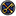•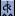•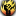•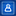•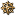•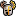•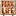••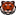•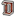•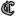•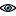••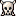•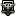•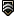•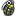•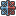•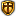•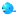••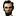•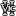•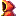•••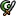•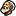•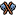•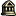•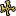•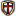Should he really be styled as Charles VIII? He styled himself as Charles II...

#### Toio

##### Field Marshal
••••••Prinz Wilhelm said:
Should he really be styled as Charles VIII? He styled himself as Charles II...

http://en.wikipedia.org/wiki/Charles_VIII_of_Sweden

I have no issue,

let me know which you prefer

...............

by the way, which dates should we choose because the 20 june 1448 he was elected king by his soldiers, yet the swedish nobles and councillors did not elect him king till november 1448

Last edited:

#### unmerged(40707)

##### Just call me Yoda in private!
Charles II then:
Referring to Karl Knutsson as Charles VIII is a later invention

But we could use this sentence for the remark.

#### Toio

##### Field Marshal
••••••YodaMaster said:
Charles II then:
Referring to Karl Knutsson as Charles VIII is a later invention

But we could use this sentence for the remark.

agree .charles II

I think we need a start date of November 1448 instead of the June one, it does not sit well with me when soldiers elect someone king before the council/state or nobles have had a say. thoughts?